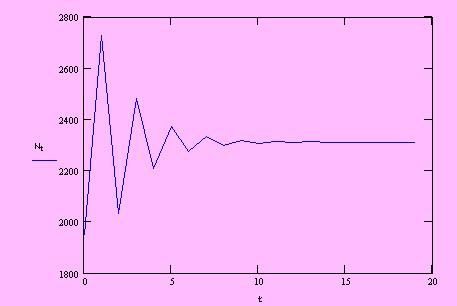Growth curve at each time step along t :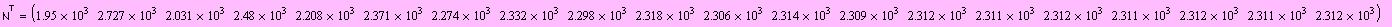which in transpose form of Nt :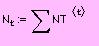Each column of the Matrix NT consists of a "one step" or an age vector of the population. The leftern most column is the initial vector N0.
The total population of each vector will To construct a graph of the population growth along the time steps 0 ... t, we have to find the total population in each vector Nt :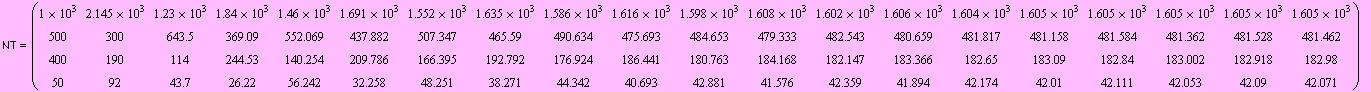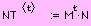Say NT is the vector of the population at time or step t, then following
Leslie equation: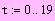Enter the time step (how many step to simulate)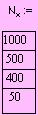Enter data for vector of initial population of each stage: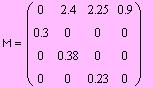Thus we have the Leslie Matrix, M :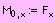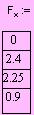Enter data for Fx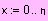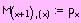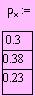Enter data for p statistic: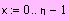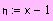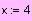Enter number of stage (x):
Since p (survival data) are located diagonally starting at first column and second row, to enter p we firstly construct a vector of x-1 dimension (n = x-1).
Also consider that Origin=0 is used in this Mathcad program.
Statistics needed for population growth simulation using Leslie model are:
F (fecundity) and p (survival) of the population in each stage of development or
age-class, and the initial population vector N. ( in some texts, m is used for F and
s for p ).
Say, x is the number of age-class, the Leslie transition matrix is Mx.x.
A Mathcad program for Leslie model population growth simulation,
by Rudy C Tarumingkeng, IPB-Bogor, Indonesia
MATRIKS PROYEKSI LESLIE --- PROGRAM MATHCAD